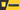### You don't know JavaScript Yet:#6 範疇鏈``````var studentName = "Suzy";

function printStudent(studentName) {
studentName = studentName.toUpperCase();
console.log(studentName);
}

printStudent("Frank");
// FRANK

printStudent(studentName);
// SUZY

console.log(studentName);
// Suzy``````

### 避免遮蔽的技巧

``````var studentName = "Suzy";

function printStudent(studentName) {
console.log(studentName);
console.log(window.studentName);
}

printStudent("Frank");
// "Frank"
// "Suzy"``````

``````var one = 1;
let notOne = 2;
const notTwo = 3;
class notThree {}

console.log(window.one);       // 1
console.log(window.notOne);    // undefined
console.log(window.notTwo);    // undefined
console.log(window.notThree);  // undefined``````

### 非法遮蔽

``````function something() {
var special = "JavaScript";
{
let special = 42;   // totally fine shadowing
// ..
}
}

function another() {
// ..
{
let special = "JavaScript";
{
var special = "JavaScript";   // Syntax Error
// ..
}
}
}``````

``````function another() {
// ..
{
let special = "JavaScript";

whatever(function callback(){
var special = "JavaScript";   // totally fine shadowing
// ..
});
}
}``````

## 函式名稱範疇

``````function askQuestion() {
// ..
}``````

``````var askQuestion = function(){
// ..
};``````

``````var askQuestion = function ofTheTeacher(){
// ..
};``````

`askQuestion`本身沒什麼問題，它將結束於外部範疇(在此例由於它存在於全域範疇，所以沒有比全域範疇更外面的範疇了)，我們這邊的重點在於`ofTheTeacher`，它也如同其他變數或者函式一般的存在嗎?顯然不是。`ofTheTeacher`作為一個識別字被宣告於它自己當中:

``````var askQuestion = function ofTheTeacher() {
console.log(ofTheTeacher);
};

// function ofTheTeacher()...

console.log(ofTheTeacher);
// ReferenceError: 'ofTheTeacher' is not defined``````

``````var askQuestion = function ofTheTeacher(){
// why is this not a duplicate declaration error?
let ofTheTeacher = "Confused, yet?";
};``````

``````var askQuestion = function ofTheTeacher() {
"use strict";
ofTheTeacher = 42;   // TypeError

//..
};

// TypeError``````

``````var askQuestion = function(){
// ..
};``````

## 箭頭函式(Arrow Functions)

``````var askQuestion = () => {
// ..
};``````

``````() => 42

id => id.toUpperCase()

(id,name) => ({ id, name })

(...args) => {
return args[args.length - 1];
};``````

## 總結

• 當函式被定義時會產生新的範疇，程式中由許多函式所結合，而這些函式的範疇彼此會產生一種階層的關係，我們稱它為範疇鏈，用來控制變數的存取。